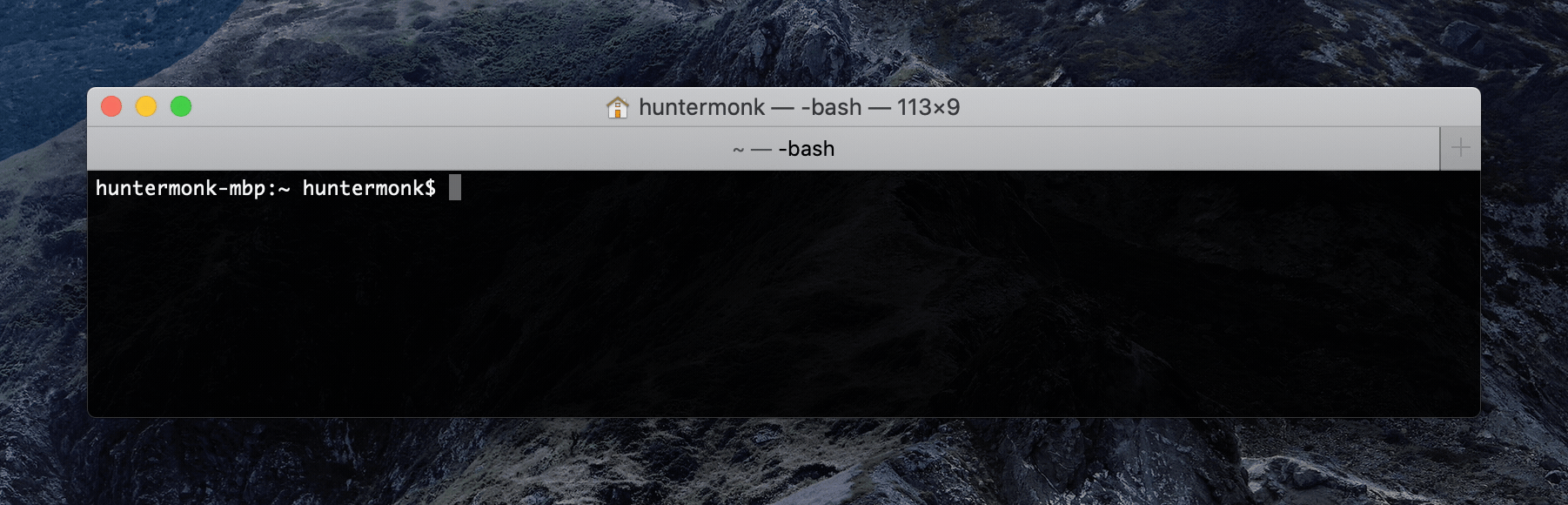In Part 1, I set a goal of 2,800 hours of work in 2021.

To make sure I don’t fall behind, I made a script to tell me if I’m on track.I don’t know Python, took me about 30 minutes.

Here’s the script:

``````print("Starting request to Harvest.")

import requests
from datetime import date
import numpy as np

<redacted>
}

today = date.today()

harvest_date_format = "%Y%m%d"
year_start = date(2021, 1, 1)
year_start_string = year_start.strftime(harvest_date_format)
today_string = today.strftime(harvest_date_format)

params = (
('from', year_start_string),
('to', today_string),
)

as_JSON = response.json()["results"]
hours = list(map(lambda x: x["total_hours"], as_JSON))
total_hours = sum(hours)
print("So far, you've worked " + str(total_hours) + " hours.")
delta = (today - year_start).days + 1
print("You are " + str(delta) + " days through the year.")
print()

year_end_hours = round((356 / delta) * total_hours, 2)
print("At this rate, you will have worked " + str(year_end_hours)  + " hours at the end of the year.")

goal_hours = 2800
hours_short = round(goal_hours - year_end_hours, 2)

goal_hours_remaining = goal_hours - total_hours
days_left = 356 - delta

year_end = date(2021, 12, 31)
workdays_left = np.busday_count(today, year_end)
goal_hours_per_day = round(goal_hours_remaining / workdays_left, 2)
print("You are " + str(hours_short) + " hours short of your goal of " + str(goal_hours) + ". To make up the difference, work " + str(goal_hours_per_day) + " hours per workday or more.")
print()
``````